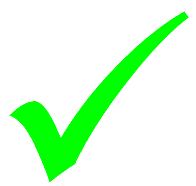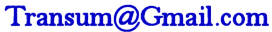# Using a Calculator Level 1

## Questions to test your ability to use a basic calculator, scientific calculator and a graphic display calculator.

##### BIDMASWorkoutBasicScientificGraphic displayDescriptionHelpMore...

This is level 1; Using a basic calculator. The questions provide practice using a calculator to obtain results accurately. You can earn a trophy if you get at least 9 questions correct.

 1. Add up all of the numbers from one to ten.1+2+3+4+5+6+7+8+9+102. Multiply together all of the even numbers from four to fourteen.3. There were 55000 people at the game. 33000 of them were supporting United. How many of them were not supporting United?4. Divide 101.931 by 1.6715. Find 37% of 480006. Calculate how many seconds there are in 13 full days.7. How many inches are in one third of a mile if there are 12 inches in a foot, three feet in a yard and 1760 yards in a mile?8. For every 40 points you earn on your restaurant discount card you are given a free desert voucher. You earn 1 point for every £1 you spend and last month you visited the restaurant 10 times spending £25 on each visit. How many desert vouchers did you receive?9. A Trantours bus can hold no more than 41 people. How many buses will be needed to transport 719 people to a concert?10. Evaluate 36 × 29 + 99911. Evaluate 47 × (588 − 121)12. Evaluate (308 − 221) − (565 − 121)Check

This is Basic calculator. You can also try:
BIDMAS Calculator Workout Scientific calculator Graphic display calculator

## Instructions

Try your best to answer the questions above. Type your answers into the boxes provided leaving no spaces. As you work through the exercise regularly click the "check" button. If you have any wrong answers, do your best to do corrections but if there is anything you don't understand, please ask your teacher for help.

When you have got all of the questions correct you may want to print out this page and paste it into your exercise book. If you keep your work in an ePortfolio you could take a screen shot of your answers and paste that into your Maths file.

## Transum.org

This web site contains over a thousand free mathematical activities for teachers and pupils. Click here to go to the main page which links to all of the resources available.

Please contact me if you have any suggestions or questions.## More Activities:

Mathematicians are not the people who find Maths easy; they are the people who enjoy how mystifying, puzzling and hard it is. Are you a mathematician?

Comment recorded on the 16 March 'Starter of the Day' page by Mrs A Milton, Ysgol Ardudwy:

"I have used your starters for 3 years now and would not have a lesson without one! Fantastic way to engage the pupils at the start of a lesson."

Comment recorded on the 8 May 'Starter of the Day' page by Mr Smith, West Sussex, UK:

"I am an NQT and have only just discovered this website. I nearly wet my pants with joy.
To the creator of this website and all of those teachers who have contributed to it, I would like to say a big THANK YOU!!! :)."

#### Tower of HanoiMove the pieces of the tower from one place to another in the minimum number of moves. This puzzle was invented in 1883 but is still as captivating today as it was all those years ago.

There are answers to this exercise but they are available in this space to teachers, tutors and parents who have logged in to their Transum subscription on this computer.

A Transum subscription unlocks the answers to the online exercises, quizzes and puzzles. It also provides the teacher with access to quality external links on each of the Transum Topic pages and the facility to add to the collection themselves.

Subscribers can manage class lists, lesson plans and assessment data in the Class Admin application and have access to reports of the Transum Trophies earned by class members.

If you would like to enjoy ad-free access to the thousands of Transum resources, receive our monthly newsletter, unlock the printable worksheets and see our Maths Lesson Finishers then sign up for a subscription now:

Subscribe

## Go Maths

Learning and understanding Mathematics, at every level, requires learner engagement. Mathematics is not a spectator sport. Sometimes traditional teaching fails to actively involve students. One way to address the problem is through the use of interactive activities and this web site provides many of those. The Go Maths page is an alphabetical list of free activities designed for students in Secondary/High school.

## Maths Map

Are you looking for something specific? An exercise to supplement the topic you are studying at school at the moment perhaps. Navigate using our Maths Map to find exercises, puzzles and Maths lesson starters grouped by topic.

## Teachers

If you found this activity useful don't forget to record it in your scheme of work or learning management system. The short URL, ready to be copied and pasted, is as follows:

Do you have any comments? It is always useful to receive feedback and helps make this free resource even more useful for those learning Mathematics anywhere in the world. Click here to enter your comments.For All:

## Description of Levels

BIDMAS - Learn about the order of operations.

Calculator Workout - Examples of all the major calculator operations.

CloseLevel 1 - Basic calculator quiz.

Level 2 - Scientific calculator quiz.

Level 3 - Graphic display calculator quiz.

More on this topic including lesson Starters, visual aids and investigations.

Answers to this exercise are available lower down this page when you are logged in to your Transum account. If you don’t yet have a Transum subscription one can be very quickly set up if you are a teacher, tutor or parent.

## Examples

Click on a link below to see an example and an animated key-press diagram of a calculator.

Skill 1: Basic Operations e.g. Calculate the value of $$9+4−7$$

Skill 2: Using Brackets e.g. Calculate the value of $$5(9-4)$$

Skill 3: Percentage of a quantity e.g. What is $$24% of 1800$$?

Skill 4: One quantity as a percentage of another e.g. What is $$45$$ as a percentage of $$150$$?

Skill 5: Squares and square roots e.g. Calculate the value of $$\sqrt{5^2 + 12^2}$$

Skill 6: Indices e.g. $$(3^3 + 2^4)^2$$

Skill 7: Cube roots e.g. Find the value of $$\sqrt{27}$$

Skill 8: Standard Form e.g. Express $$236731$$ in standard form correct to 3 significant figures.

Skill 9: Areas of circles e.g. [Give all answers correct to three significant figures]. e.g. Find the area of a circle with a radius of 9cm.

Skill 10: Fraction calculations e.g. Find the value of $$3\frac13 + 2\frac25$$

Skill 11: Trigonometry e.g. Evaluate $$21 \sin34°$$ to three significant figures 1.

Skill 12: Degrees (or Hours), Minutes and Seconds e.g. Convert the angle 16°35'40" to its decimal equivalent.

Skill 13: Using Variables e.g. If $$A = 1.62$$, $$B = 9.87$$ and $$C = 5.62$$
evaluate $$AB+BC−AC$$.

Skill 14: Combinations e.g. How many different groups of 3 can be selected from 8?

Skill 15: Permutations e.g. In how many different ways could 1st, 2nd and 3rd place prizes can be awarded to 3 of 8 competitors?

Don't wait until you have finished the exercise before you click on the 'Check' button. Click it often as you work through the questions to see if you are answering them correctly. You can double-click the 'Check' button to make it float at the bottom of your screen.

Answers to this exercise are available lower down this page when you are logged in to your Transum account. If you don’t yet have a Transum subscription one can be very quickly set up if you are a teacher, tutor or parent.

Close